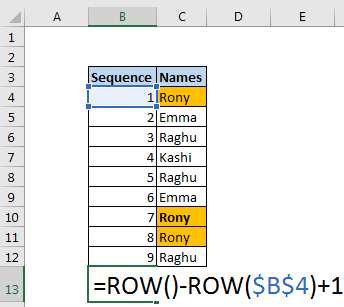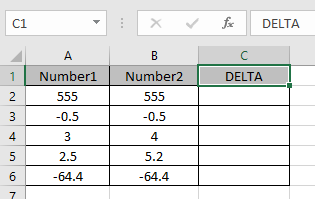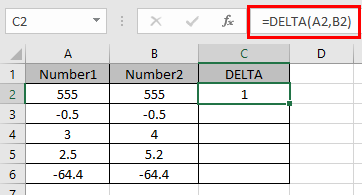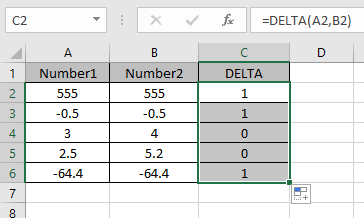# How to use the DELTA Function in Excel

In simple words, this function only returns 1 when the values are an exact match with each other or else 0.

DELTA function returns 1 or 0 stated if the given two numbers are equal or not.

Syntax:

=DELTA (number1, [number2])

Number1 : first value.
Number2 : second value.

Let’s understand this function using it in an example.Here we performed 2 operations in the DELTA function.
4 + 2 = 6
3 * 2 = 6
As you can see from the above operations result is equal so the function returns 1.
Now let’s use the function giving argument as cell reference.Here we have some numbers in Column 1 & 2. ANd we need to find the exact number match.
Use the formula:

=DELTA(A2,B2)

A2 : first value given in as cell reference.
B2 : second value given in as cell reference.As you can see the DELTA function returns 1 only if both values are exact or equal match.

Now copy the formula in other cells using Ctrl + D shortcut key.Hope understood how to use the DELTA function in Excel. Explore mkore articles on Excel logic function here. Fell free to state your query in the comment box below. we will help you.

Popular Articles

Edit a dropdown list

If with conditional formatting

If with wildcards

Vlookup by date

Terms and Conditions of use

The applications/code on this site are distributed as is and without warranties or liability. In no event shall the owner of the copyrights, or the authors of the applications/code be liable for any loss of profit, any problems or any damage resulting from the use or evaluation of the applications/code.